# CEV模型下鲁棒最优投资和超额损失再保险问题研究Research on Robust Optimal Investment and Excess-of-Loss Reinsurance under CEV Model

DOI: 10.12677/SA.2018.75058, PDF, HTML, XML, 下载: 523  浏览: 708

Abstract: In this paper, we investigate an optimal investment and excess-of-loss reinsurance strategy for an ambiguity-averse insurer (AAI). The financial market consists of one risk-free asset and one risky asset whose price is modeled by a constant elasticity of variance (CEV) model. The insurer can purchase excess-of-loss reinsurance and invest in the financial market. The surplus process of the insurer is approximated by a Brownian motion with drift. The objective is to maximize the minimal expected exponential utility function of the insurer's terminal wealth. By using the dynamic programming approach, we solve the Hamilton-Jacobi-Bellman (HJB) equation and derive the closed form expression of the optimal strategy and the corresponding value function for exponential utility function. Finally, we present numerical examples to illustrate the effects of model parameters on the optimal investment and reinsurance strategies.

1. 引言

2. 模型介绍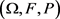是满足通常条件的完备概率空间， ${\left\{{F}_{t}\right\}}_{0\le t\le T}$ 表示概率空间到时刻t的域流，其中 $T>0$ 为给定的正的有限投资时间； ${F}_{t}$ 代表到时间t的可用信息。下面介绍的所有随机过程都是这个空间中的适应过程。

$R\left(t\right)={c}_{0}+ct-\underset{i=1}{\overset{N\left(t\right)}{\sum }}{Y}_{i},$

${R}^{\left(a\right)}\left(t\right)={c}_{0}+{c}^{\left(a\right)}t-\underset{i=1}{\overset{N\left(t\right)}{\sum }}{Y}_{i}^{\left(q\right)},$

${c}^{\left(a\right)}=\left(1+\eta \right)\lambda {\mu }_{1}-\left(1+\theta \right)\lambda \left({\mu }_{1}-E{Y}_{i}^{\left(a\right)}\right)=\left(\eta -\theta \right)\lambda {\mu }_{1}+\lambda \left(1+\theta \right)E{Y}_{i}^{\left(a\right)},$

$\theta$ 为再保险公司的安全负荷，并且 $\theta >\eta$ 。为了方便，盈余过程 ${R}^{\left(a\right)}\left(t\right)$ 可近似为扩散模型

$\text{d}{R}^{\left(a\right)}\left(t\right)=\lambda \left[\theta \mu \left(a\right)+\left(\eta -\theta \right){\mu }_{1}\right]\text{d}t+\sqrt{\lambda }\sigma \left(a\right)\text{d}{W}_{0}\left(t\right),$

$\begin{array}{l}\mu \left(a\right)=E{Y}_{i}^{\left(a\right)}={\int }_{0}^{a}\left(1-F\left(u\right)\right)\text{d}u={\int }_{0}^{a}\stackrel{¯}{F}\left(u\right)\text{d}u,\\ {\sigma }^{2}\left(a\right)=E{\left({Y}_{i}^{\left(a\right)}\right)}^{2}={\int }_{0}^{a}2u\left(1-F\left(u\right)\right)\text{d}u={\int }_{0}^{a}2u\stackrel{¯}{F}\left(u\right)\text{d}u,\end{array}$

$\left\{\begin{array}{l}\text{d}B\left(t\right)=rB\left(t\right)\text{d}t,\text{\hspace{0.17em}}t\in \left[0,T\right],\\ B\left(0\right)={b}_{0}>0,\end{array}$

$\left\{\begin{array}{l}\text{d}S\left(t\right)=S\left(t\right)\left[\mu \text{d}t+\sigma {S}^{\beta }\left(t\right)\text{d}{W}_{1}\left(t\right)\right],\text{\hspace{0.17em}}\text{\hspace{0.17em}}t\in \left[0,T\right],\\ S\left(0\right)={s}_{0}>0,\end{array}$

${X}^{\text{π}}\left(t\right)$ 表示t时刻保险公司的财富， $a\left(t\right)\in \left[0,D\right]$ 表示t时刻的超额损失自留水平， ${\text{π}}_{\text{1}}\left(t\right)$ 表示投资到风险资产中的数额。 $\text{π}={\left(a\left(t\right),{\text{π}}_{1}\left(t\right)\right)}_{t\in \left[0,T\right]}$ 表示一个交易策略。在策略 $\text{π}$ 下，财富过程 ${\left\{{X}^{\pi }\left(t\right)\right\}}_{t\in \left[0,T\right]}$ 表示为

$\begin{array}{c}\text{d}{X}^{\text{π}}\left(t\right)=\left[r{X}^{\pi }\left(t\right)+\left(\mu -r\right){\text{π}}_{1}\left(t\right)+\lambda \theta \mu \left(a\right)+\lambda \left(\eta -\theta \right){\mu }_{1}\right]\text{d}t\\ \text{\hspace{0.17em}}\text{\hspace{0.17em}}+\sqrt{\lambda }\sigma \left(a\right)\text{d}{W}_{0}\left(t\right)+{\text{π}}_{1}\left(t\right)\sigma {S}^{\beta }\left(t\right)\text{d}{W}_{1}\left(t\right)\end{array}$ (1)

3. 效用函数下的鲁棒问题

$U\left(x\right)=-\frac{1}{\gamma }\mathrm{exp}\left\{-\gamma x\right\},$ (2)

$\underset{\text{π}\in \prod }{\mathrm{sup}}E\left[U\left({X}^{\text{π}}\left(T\right)\right)\right]=\underset{\text{π}\in \prod }{\mathrm{sup}}E\left[-\frac{1}{\gamma }\mathrm{exp}\left\{-\gamma {X}^{\text{π}}\left(T\right)\right\}\right],$ (30

${\Lambda }^{\omega }\left(t\right):=\mathrm{exp}\left\{-{\int }_{0}^{t}{\omega }_{1}\left(s\right)\text{d}{W}_{0}\left(s\right)-\frac{\text{1}}{\text{2}}{\int }_{0}^{t}{\omega }_{1}^{2}\left(s\right)\text{d}s-{\int }_{0}^{t}{\omega }_{2}\left(s\right)\text{d}{W}_{1}\left(s\right)-\frac{\text{1}}{\text{2}}{\int }_{\text{0}}^{t}{\omega }_{2}^{2}\left(s\right)\text{d}s\right\}.$ (4)

$\text{d}{W}_{0}^{Q}\left(t\right)=\text{d}{W}_{0}\left(t\right)+{\omega }_{1}\left(t\right)\text{d}t,\text{\hspace{0.17em}}\text{\hspace{0.17em}}\text{d}{W}_{1}^{Q}\left(t\right)=\text{d}{W}_{1}\left(t\right)+{\omega }_{2}\left(t\right)\text{d}t.$ (5)

CEV模型描述为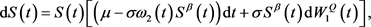(6)

$\begin{array}{c}\text{d}{X}^{\text{π}}\left(t\right)=\left[r{X}^{\text{π}}\left(t\right)+\left(\mu -r\right){\text{π}}_{1}\left(t\right)+\lambda \theta \mu \left(a\right)+\lambda \left(\eta -\theta \right){\mu }_{1}-{\omega }_{1}\left(t\right)\sqrt{\lambda }\sigma \left(a\right)\\ \text{\hspace{0.17em}}\text{\hspace{0.17em}}-\sigma {\omega }_{2}\left(t\right){\text{π}}_{1}\left(t\right){S}^{\beta }\left(t\right)\right]\text{d}t+\sqrt{\lambda }\sigma \left(a\right)\text{d}{W}_{0}^{Q}\left(t\right)+{\text{π}}_{1}\left(t\right)\sigma {S}^{\beta }\left(t\right)\text{d}{W}_{1}^{Q}\left(t\right).\end{array}$ (7)

1) $\left(a\left(t\right),{\text{π}}_{1}\left(t\right)\right)$ 是可测的；

2) $a\left(t\right)\in \left[0,D\right]$${\text{π}}_{\text{1}}\left(t\right)\in \left[0,+\infty \right)$${E}^{{Q}^{*}}\left[{\int }_{0}^{T}{\text{π}}_{1}{\left(t\right)}^{2}{S}^{2\beta }\text{d}t\right]<+\infty$

3) $\forall \left(t,x,s\right)\in \left[0,T\right]×R×{R}_{+}$ ，SDE (7)有唯一(强)解 $\left\{{X}^{\text{π}}\left(t\right):t\in \left[0,T\right]\right\}$${E}_{t,x,s}^{{Q}^{*}}\left[U\left({X}^{\text{π}}\left(T\right)\right)\right]<\infty$${Q}^{*}$ 是描述最坏情况下的备选模型。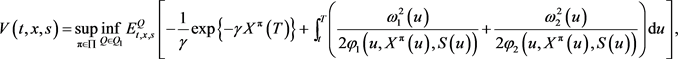(8)

$\frac{\text{1}}{\text{2}}{\omega }_{\text{1}}^{\text{2}}\left(t\right)\text{d}t+\frac{1}{2}{\omega }_{2}^{2}\left(t\right)\text{d}t.$

${\phi }_{i}\left(t,x,s\right)=-\frac{{\beta }_{i}}{\gamma V\left(t,x,s\right)},i=1,2,$ (9)

$\underset{\text{π}\in \prod }{\mathrm{sup}}\underset{Q\in {Q}_{1}}{\mathrm{inf}}\left\{{Α}^{\text{π},\omega }V+\frac{{\omega }_{1}^{2}}{2{\phi }_{1}}+\frac{{\omega }_{2}^{2}}{2{\phi }_{2}}\right\}=0$ (10)

$\begin{array}{c}{Α}^{\text{π},\omega }V={V}_{t}+\left[rx+\left(\mu -r\right){\text{π}}_{1}+\lambda \theta \mu \left(a\right)+\lambda \left(\eta -\theta \right){\mu }_{1}-{\omega }_{1}\sqrt{\lambda }\sigma \left(a\right)-\sigma {\omega }_{2}{\text{π}}_{1}{s}^{\beta }\right]{V}_{x}\\ \text{\hspace{0.17em}}\text{\hspace{0.17em}}+\frac{\text{1}}{\text{2}}\left({\sigma }^{\text{2}}{\text{π}}_{\text{1}}^{\text{2}}{s}^{2\beta }+\lambda \sigma {\left(a\right)}^{2}\right){V}_{xx}+s\left(\mu -\sigma {\omega }_{2}{s}^{\beta }\right){V}_{s}+\frac{\text{1}}{\text{2}}{\sigma }^{2}{s}^{2\beta +2}{V}_{ss}+{\sigma }^{2}{s}^{2\beta +1}{\text{π}}_{1}{V}_{xs}.\end{array}$ (11)

$V\left(t,x,s\right)=-\frac{\text{1}}{\gamma }\mathrm{exp}\left\{-\gamma \left[x{\text{e}}^{r\left(T-t\right)}+h\left(t,s\right)+g\left(t\right)\right]\right\}\text{.}$ (12)

$\begin{array}{l}{V}_{t}=-\gamma \left[{g}_{t}+{h}_{t}-r{\text{e}}^{r\left(T-t\right)}x\right]V,\text{\hspace{0.17em}}\text{\hspace{0.17em}}{V}_{x}=-\gamma {\text{e}}^{r\left(T-t\right)}V,\text{\hspace{0.17em}}\text{\hspace{0.17em}}{V}_{s}=-\gamma {h}_{s}V,\\ {V}_{xx}={\gamma }^{2}{\text{e}}^{2r\left(T-t\right)}V,\text{\hspace{0.17em}}\text{\hspace{0.17em}}{V}_{ss}=-\gamma \left[{h}_{ss}-\gamma {h}_{s}^{2}\right]V,\text{\hspace{0.17em}}\text{\hspace{0.17em}}{V}_{xs}={\gamma }^{2}{\text{e}}^{r\left(T-t\right)}{h}_{s}V.\end{array}$ (13)

${\omega }_{\text{1}}^{\text{*}}={\beta }_{\text{1}}\sqrt{\lambda }\sigma \left(a\right){\text{e}}^{r\left(T-t\right)},\text{\hspace{0.17em}}\text{\hspace{0.17em}}\text{\hspace{0.17em}}{\omega }_{\text{2}}^{\text{*}}={\beta }_{\text{2}}\sigma {s}^{\beta }\left({\text{π}}_{1}{\text{e}}^{r\left(T-t\right)}+s{h}_{s}\right).$ (14)

${\text{π}}_{\text{1}}^{\ast }=\frac{\mu -r-{\sigma }^{2}{s}^{2\beta +1}{h}_{s}\left(\gamma +{\beta }_{2}\right)}{{\sigma }^{2}{s}^{2\beta }{\text{e}}^{r\left(T-t\right)}\left(\gamma +{\beta }_{2}\right)}.$ (15)

$\left[\theta \gamma {\text{e}}^{r\left(T-t\right)}-\left({\gamma }^{2}{\text{e}}^{2r\left(T-t\right)}+\gamma {\beta }_{1}{\text{e}}^{2r\left(T-t\right)}\right)a\left(t\right)\right]\lambda \stackrel{¯}{F}\left(a\left(t\right)\right)=0.$ (16)

${a}^{\ast }\left(t\right)=\frac{\theta }{\gamma +{\beta }_{1}}{\text{e}}^{-r\left(T-t\right)}.$ (17)

$\begin{array}{l}{g}_{t}+\lambda \left(\eta -\theta \right){\mu }_{1}{\text{e}}^{r\left(T-t\right)}+\lambda \theta {\text{e}}^{r\left(T-t\right)}{\int }_{\text{0}}^{\frac{\theta {\text{e}}^{-r\left(T-t\right)}}{\gamma +{\beta }_{1}}}\stackrel{¯}{F}\left(u\right)\text{d}u\\ -\frac{\text{1}}{\text{2}}\left(\lambda \gamma {\text{e}}^{2r\left(T-t\right)}+{\beta }_{1}\lambda {\text{e}}^{2r\left(T-t\right)}\right){\int }_{0}^{\frac{\theta {\text{e}}^{-r\left(T-t\right)}}{\gamma +{\beta }_{1}}}2u\stackrel{¯}{F}\left(u\right)\text{d}u=0,\\ {h}_{t}+sr{h}_{s}+\frac{\gamma }{2}{\sigma }^{2}{s}^{2\beta +2}{h}_{ss}+\frac{{\left(\mu -r\right)}^{2}}{2\left(\gamma +{\beta }_{2}\right){\sigma }^{2}{s}^{2\beta }}=0.\end{array}$ (18)

$g\left(t\right)=\frac{\lambda \left(\eta -\theta \right){\mu }_{1}}{r}\left({\text{e}}^{r\left(T-t\right)}-1\right)+{\int }_{t}^{T}f\left(s\right)\text{d}s,$ (19)

$f\left(t\right)=\lambda \theta {\text{e}}^{r\left(T-t\right)}{\int }_{0}^{\frac{\theta {\text{e}}^{-r\left(T-t\right)}}{\gamma +{\beta }_{1}}}\stackrel{¯}{F}\left(u\right)\text{d}u-\frac{1}{2}\left(\lambda \gamma {\text{e}}^{2r\left(T-t\right)}+{\beta }_{1}\lambda {\text{e}}^{2r\left(T-t\right)}\right){\int }_{0}^{\frac{\theta {\text{e}}^{-r\left(T-t\right)}}{\gamma +{\beta }_{1}}}2u\stackrel{¯}{F}\left(u\right)\text{d}u.$

$h\left(t,s\right)=A\left(t\right)+B\left(t\right){s}^{-2\beta },$ (20)

$\begin{array}{l}{A}_{t}+\gamma {\sigma }^{2}\beta \left(2\beta +1\right)B\left(t\right)=0,\\ {B}_{t}-2r\beta B\left(t\right)+\frac{{\left(\mu -r\right)}^{2}}{2\left(\gamma +{\beta }_{2}\right){\sigma }^{2}}=0.\end{array}$ (21)

 (22)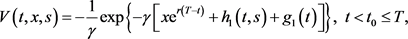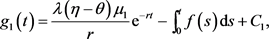(23)

$\begin{array}{c}{h}_{1}\left(t,s\right)=\frac{\left(2\beta +1\right){\left(\mu -r\right)}^{2}}{8{r}^{2}\beta \left(\gamma +{\beta }_{2}\right)}{\text{e}}^{2r\beta t}{C}_{2}-\frac{\left(2\beta +1\right){\left(\mu -r\right)}^{2}}{4r\left(\gamma +{\beta }_{2}\right)}t+{C}_{3}\\ \text{\hspace{0.17em}}\text{\hspace{0.17em}}+\left[{C}_{4}-{C}_{5}\frac{{\left(\mu -r\right)}^{2}}{4r\beta \left(\gamma +{\beta }_{2}\right){\sigma }^{2}}{\text{e}}^{-2r\beta t}\right]{s}^{-2\beta },\end{array}$ (24)

$V\left(t,x,s\right)=-\frac{\text{1}}{\gamma }\mathrm{exp}\left\{-\gamma \left[x{\text{e}}^{r\left(T-t\right)}+{h}_{\text{2}}\left(t,s\right)+{g}_{\text{2}}\left(t\right)\right]\right\},\text{\hspace{0.17em}}\text{\hspace{0.17em}}{t}_{0}\le t\le T,$

$\begin{array}{l}{h}_{2}\left(t,s\right)=h\left(t,s\right),\\ {g}_{2}\left(t\right)=\frac{\lambda \eta {\mu }_{1}}{r}\left({\text{e}}^{r\left(T-t\right)}-1\right)-\frac{\lambda {\mu }_{2}^{2}}{4r}\left(\gamma +{\beta }_{1}\right)\left({\text{e}}^{2r\left(T-t\right)}-1\right).\end{array}$ (25)

${h}_{1}\left({t}_{0},s\right)+{g}_{1}\left({t}_{0}\right)={h}_{2}\left({t}_{0},s\right)+{g}_{2}\left({t}_{0}\right),$

${C}_{1}={g}_{2}\left({t}_{0}\right)-\frac{\lambda \left(\eta -\theta \right){\mu }_{1}}{r}{\text{e}}^{-r{t}_{0}}+{\int }_{0}^{{t}_{0}}f\left(s\right)\text{d}s,$

${C}_{2}=\frac{\left(2\beta +1\right){\left(\mu -r\right)}^{2}}{8{r}^{2}\beta \left(\gamma +{\beta }_{2}\right)}{\text{e}}^{-2r\beta T},\text{\hspace{0.17em}}\text{\hspace{0.17em}}{C}_{3}=-\frac{\left(2\beta +1\right){\left(\mu -r\right)}^{2}}{8{r}^{2}\beta \left(\gamma +{\beta }_{2}\right)}+\frac{\left(2\beta +1\right){\left(\mu -r\right)}^{2}}{4r\left(\gamma +{\beta }_{2}\right)}T,$

${C}_{4}=\frac{{\left(\mu -r\right)}^{2}}{4r\beta \left(\gamma +{\beta }_{2}\right){\sigma }^{2}},\text{\hspace{0.17em}}\text{\hspace{0.17em}}{C}_{5}=\frac{{\left(\mu -r\right)}^{2}}{4r\beta \left(\gamma +{\beta }_{2}\right){\sigma }^{2}}{\text{e}}^{-2r\beta T}.$

1) 如果 $D\left(\gamma +{\beta }_{1}\right)\ge \theta$

${\text{π}}_{\text{1}}^{\ast }\left(t\right)=\frac{\mu -r+2\beta {\sigma }^{2}\left(\gamma +{\beta }_{2}\right)B\left(t\right)}{{\sigma }^{2}{s}^{2\beta }{\text{e}}^{r\left(T-t\right)}\left(\gamma +{\beta }_{2}\right)},\text{\hspace{0.17em}}\text{\hspace{0.17em}}{a}^{\ast }\left(t\right)=\frac{\theta }{\gamma +{\beta }_{1}}{\text{e}}^{-r\left(T-t\right)},\text{\hspace{0.17em}}\text{\hspace{0.17em}}0\le t\le T,$

$J\left(t,x,s\right)=-\frac{\text{1}}{\gamma }\mathrm{exp}\left\{-\gamma \left[x{\text{e}}^{r\left(T-t\right)}+h\left(t,s\right)+g\left(t\right)\right]\right\},\text{\hspace{0.17em}}\text{\hspace{0.17em}}\text{0}\le t\le T,$ (26)

2) 如果 $D\left(\gamma +{\beta }_{1}\right)<\theta$

$J\left(t,x,s\right)=\left\{\begin{array}{l}-\frac{1}{\gamma }\mathrm{exp}\left\{-\gamma \left[x{\text{e}}^{r\left(T-t\right)}+h\left(t,s\right)+{g}_{1}\left(t\right)\right]\right\},t<{t}_{0}\le T,\\ -\frac{1}{\gamma }\mathrm{exp}\left\{-\gamma \left[x{\text{e}}^{r\left(T-t\right)}+h\left(t,s\right)+{g}_{2}\left(t\right)\right]\right\},{t}_{0}\le t (27)

(1) ${\text{π}}^{\ast }$ 是可行策略；

(2) ${E}^{{Q}^{*}}\left[{\mathrm{sup}}_{t\in \left[0,T\right]}{|J\left(t,{X}^{{\text{π}}^{*}}\left(t\right),S\left(t\right)\right)|}^{4}\right]<\infty$

(3) ${E}^{{Q}^{*}}\left[{\mathrm{sup}}_{t\in \left[0,T\right]}{|\frac{{\omega }_{1}^{*}{\left(t\right)}^{2}}{2{\phi }_{1}\left(t,{X}^{{\text{π}}^{*}}\left(t\right),S\left(t\right)\right)}+\frac{{\omega }_{2}^{*}{\left(t\right)}^{2}}{2{\phi }_{2}\left(t,{X}^{{\text{π}}^{*}}\left(t\right),S\left(t\right)\right)}|}^{2}\right]<\infty$

$\begin{array}{c}{X}^{{\text{π}}^{*}}\left(t\right)={\text{e}}^{rt}{X}^{{\text{π}}^{*}}\left(0\right)+{\int }_{0}^{t}{\text{e}}^{-r\left(u-t\right)}\left[\left(\mu -r\right){\text{π}}_{1}^{*}\left(u\right)+\lambda \theta \mu \left({a}^{*}\right)\\ \text{\hspace{0.17em}}\text{\hspace{0.17em}}+\lambda \left(\eta -\theta \right){\mu }_{1}-{\omega }_{1}^{*}\left(u\right)\sqrt{\lambda }\sigma \left({a}^{*}\right)-\sigma {\omega }_{2}^{\ast }\left(u\right){\text{π}}_{1}^{*}\left(u\right){s}^{\beta }\left(u\right)\right]\text{d}u\\ \text{\hspace{0.17em}}\text{\hspace{0.17em}}+{\int }_{0}^{t}{\text{e}}^{-r\left(u-t\right)}\sqrt{\lambda }\sigma \left({a}^{*}\right)\text{d}{W}_{0}^{{Q}^{*}}\left(u\right)+{\int }_{0}^{t}{\text{e}}^{-r\left(u-t\right)}{\text{π}}_{1}^{*}\left(u\right)\sigma {s}^{\beta }\left(u\right)\text{d}{W}_{1}^{{Q}^{*}}\left(u\right).\end{array}$ (28)

$\begin{array}{l}{|J\left(t,{X}^{{\text{π}}^{*}}\left(t\right),S\left(t\right)\right)|}^{4}=\frac{\text{1}}{{\gamma }^{\text{4}}}\mathrm{exp}\left\{-4\gamma \left[{\text{e}}^{r\left(T-t\right)}{X}^{{\text{π}}^{*}}\left(t\right)+A\left(t\right)+B\left(t\right){s}^{-2\beta }+g\left(t\right)\right]\right\}\\ \le {N}_{1}\mathrm{exp}\left\{-4\gamma {\text{e}}^{r\left(T-t\right)}{X}^{{\text{π}}^{*}}\left(t\right)\right\}\\ \le {N}_{2}\mathrm{exp}\left\{-4\gamma \left(-{\int }_{0}^{t}{\text{e}}^{-r\left(u-T\right)}\sigma {\omega }_{2}^{*}\left(u\right){\text{π}}_{1}^{*}\left(u\right){s}^{\beta }\left(u\right)\text{d}u\\ \text{\hspace{0.17em}}\text{\hspace{0.17em}}\text{ }+{\int }_{0}^{t}{\text{e}}^{-r\left(u-T\right)}\sqrt{\lambda }\sigma \left({a}^{*}\right)\text{d}{W}_{0}^{{Q}^{*}}\left(u\right)+{\int }_{0}^{t}{\text{e}}^{-r\left(u-T\right)}{\text{π}}_{1}^{*}\left(u\right)\sigma {s}^{\beta }\left(u\right)\text{d}{W}_{1}^{{Q}^{*}}\left(u\right)\right)\right\}\\ ={N}_{2}\left({F}_{1}\left(t\right)+{F}_{2}\left(t\right)\right),\end{array}$ (29)

$\begin{array}{c}{F}_{1}\left(t\right)=\mathrm{exp}\left\{{\int }_{0}^{t}8{\gamma }^{2}{\text{e}}^{-2r\left(u-T\right)}\lambda \sigma {\left({a}^{*}\right)}^{2}\text{d}u\right\}\cdot \mathrm{exp}\left\{{\int }_{0}^{t}-8{\gamma }^{2}{\text{e}}^{-2r\left(u-T\right)}\lambda \sigma {\left({a}^{*}\right)}^{2}\text{d}u\\ \text{\hspace{0.17em}}\text{\hspace{0.17em}}+{\int }_{0}^{t}-4\gamma {\text{e}}^{-r\left(u-T\right)}\sqrt{\lambda }\sigma \left({a}^{*}\right)\text{d}{W}_{0}^{{Q}^{*}}\left(u\right)\right\}.\end{array}$ (30)

(30)中的第一部分是一个常数，第二部分是鞅。所以 ${E}^{{Q}^{*}}\left[{F}_{1}\left(t\right)\right]<\infty$ 。根据参考文献Zheng等  中的Lemma 3.6，我们知道 ${E}^{{Q}^{*}}\left[{F}_{2}\left(t\right)\right]<\infty$ 。因此，

${E}^{{Q}^{*}}\left[{\mathrm{sup}}_{t\in \left[0,T\right]}{|J\left(t,{X}^{{\pi }^{*}}\left(t\right),S\left(t\right)\right)|}^{4}\right]<\infty .$ (31)

$\begin{array}{l}{E}^{{Q}^{*}}\left[\underset{t\in \left[0,T\right]}{\mathrm{sup}}{|\frac{{\omega }_{1}^{*}{\left(t\right)}^{2}}{2{\phi }_{1}\left(t,{X}^{{\text{π}}^{*}}\left(t\right),S\left(t\right)\right)}+\frac{{\omega }_{2}^{*}{\left(t\right)}^{2}}{2{\phi }_{2}\left(t,{X}^{{\text{π}}^{*}}\left(t\right),S\left(t\right)\right)}|}^{2}\right]\\ ={E}^{{Q}^{*}}\left[\underset{t\in \left[0,T\right]}{\mathrm{sup}}{|\frac{{\omega }_{1}^{*}{\left(t\right)}^{2}\gamma }{2{\beta }_{1}}+\frac{{\omega }_{2}^{*}{\left(t\right)}^{2}\gamma }{2{\beta }_{2}}|}^{2}{|J\left(t,{X}^{{\text{π}}^{*}}\left(t\right),S\left(t\right)\right)|}^{2}\right]\\ \le {\left({E}^{{Q}^{*}}\left[\underset{t\in \left[0,T\right]}{\mathrm{sup}}{|\frac{{\omega }_{1}^{*}{\left(t\right)}^{2}\gamma }{2{\beta }_{1}}+\frac{{\omega }_{2}^{*}{\left(t\right)}^{2}\gamma }{2{\beta }_{2}}|}^{4}\right]\right)}^{1/2}{\left({E}^{{Q}^{*}}\left[\underset{t\in \left[0,T\right]}{\mathrm{sup}}{|J\left(t,{X}^{{\text{π}}^{*}}\left(t\right),S\left(t\right)\right)|}^{4}\right]\right)}^{1/2}\\ <\infty .\end{array}$ (32)

4. 总结

  Browne, S. (1995) Optimal Investment Policies for a Firm with Random Risk Process: Exponential Utility and Minimizing the Probability of Ruin. Mathematics of Operations Research, 20, 937-958. https://doi.org/10.1287/moor.20.4.937  Yang, H.L. and Zhang, L.H. (2005) Optimal Investment for Insurer with Jump-Diffusion Risk Process. Insurance Mathematics and Economics, 37, 615-634. https://doi.org/10.1016/j.insmatheco.2005.06.009  Dias, J.C. and Nunes, J.P. (2011) Pricing Real Options under the Constant Elasticity of Variance Diffusion. Journal of Futures Markets, 3, 230-250. https://doi.org/10.1002/fut.20468  Gu, A.L., Guo, X.P., Li, Z.F. and Zeng, Y. (2012) Optimal Control of Excess-of-Loss Reinsurance and Investment for Insurers under a CEV Model. Insurance Mathematics and Economics, 51, 674-684. https://doi.org/10.1016/j.insmatheco.2012.09.003  Gu, M.D., Yang, Y.P., Li, S.D. and Zhang, J.Y. (2010) Constant Elasticity of Variance Model for Proportional Reinsurance and Investment Strategies. Insurance Mathematics and Economics, 46, 580-587. https://doi.org/10.1016/j.insmatheco.2010.03.001  Wang, Y.J., Rong, X.M. and Zhao, H. (2018) Optimal Investment Strat-egies for an Insurer and a Reinsurer with a Jump Diffusion Risk Process under the CEV Model. Journal of Computational and Applied Mathematics, 328, 414-431. https://doi.org/10.1016/j.cam.2017.08.001  Zhang, X. and Siu, T.K. (2009) Optimal Investment and Reinsurance of an Insurer with Model Uncertainty. Insurance Mathematics and Economics, 45, 81-88. https://doi.org/10.1016/j.insmatheco.2009.04.001  Branger, N. and Larsen, L.S. (2013) Robust Portfolio Choice with Un-certainty about Jump and Diffusion Risk. Journal of Banking and Finance, 37, 5036-5047. https://doi.org/10.1016/j.jbankfin.2013.08.023  Wang, P. and Li, Z.F. (2018) Robust Optimal Investment Strategy for an AAM of DC Pension Plans with Stochastic Interest Rate and Stochastic Volatility. Insurance Mathematics and Economics, 80, 67-83. https://doi.org/10.1016/j.insmatheco.2018.03.003  Zheng, X.X., Zhou, J.M. and Sun, Z.Y. (2016) Robust Optimal Portfolio and Proportional Reinsurance for an Insurer under a CEV Model. Insurance Mathematics and Economics, 67, 77-87. https://doi.org/10.1016/j.insmatheco.2015.12.008  Maenhout, P.J. (2006) Robust Portfolio Rules and Detection-Error Probabilities for a Mean-Reverting Risk Premium. Journal of Economic Theory, 128, 136-163. https://doi.org/10.1016/j.jet.2005.12.012  Kraft, H. (2004) Optimal Portfolios with Stochastic Interest Rates and Defaultable Assets. Springer-Verlag, Berlin. https://doi.org/10.1007/978-3-642-17041-6